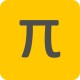It ain’t rocket science, but quadcopter science still isn’t simple. I’ve got my calibration wrong for the accelerometer. I’d omitted the fact that if Phoebe is static, depending on how she is leaning, gravity can be shared across 3 axes.  Don’t get me wrong, I’ve had this covered for ages converting from Phoebe’s axes acceleration to earth axes, but not for the accelerometer calibration. Critically, this means the trend lines for acceleration vs. temperature calibration are wrong leading to drift, and odd scaling between axes – i.e. everything I’m seeing going wrong right now.

Here’s a diagram of Phoebe’s sensor readings from the rear in red; her left side is tilted down by a negative roll angle θ.  Her pitch angle is 0 (horizontal). The only force is gravity shown in green as a positive value which is how the sensors measure it.  Hence the combination of the the qay and qaz sensors combines together to make up 1g of gravity.  Likewise, a different combination of qay and qaz cancel each other out to make 0g horizontal acceleration. The same applies to the X-axis and pitch angles, but using the Y-axis / roll angles is easier to visualize, and she does have a cute bum!

The result is the following ‘matrix’ for conversion from Phoebe’s accelerometer readings to earth coordinates:

```eax = qax * cos(pitch) + qaz * sin(pitch)
eay = qay * cos(roll) + qaz * sin(roll)
eaz = qaz * cos(pitch) * cos(roll) - qax * sin(pitch) - qay * sin(roll)```

If Phoebe is not accelerating, then the only force is vertical gravity, so eax and eay are both 0g and eaz is 1g. So assuming the accelerometer readings are perfect, and she’s sitting on the 21° tilt test rig with the fore-aft axis horizontal…

```0 = qax * cos(0°) + qaz * sin(0°)
0 = qay * cos(-21°) + qaz * sin(-21°)
1 = qaz * cos(-21°) - qay * sin(-21°)```

The calibration then involves calculating the offset and gain to convert accelerometer raw reading to ‘perfect’ qax, qay and qaz readings so that conversion to the earth axes leads to desired (0, 0, 1) as above Accurate calibrated Z axis offset and gain already exists from the previous calibration testing.  So together the equations collapse down to

```0 = qax
0 = qaygain(qayraw - qayoffset) * cos(-21°) + qaz * sin(-21°)
1 = qaz * cos(-21°) - qaygain(qayraw - qayoffset)sin(-21°)```

The Y offset can be calculated from the raw Y sensor readings with port and starboard pointing down on the rig – any difference between the two gives the offset:

`qayoffset = (qayport down + qaystarboard down) / 2`

As a result, the calculations collapse further to…

```qaygain(qayraw - qayoffset) = -qaz * tan(-21°)
qaygain(qayraw - qayoffset) = (qaz * cos(-21°) - 1) / sin(-21°)```

And now I’m bemused / confused.  qayoffset is known which means I have, what seems to me, 2 none equivalent equations for the qazgain Help!  Where have I gone wrong?

## 4 thoughts on “Math(s) help please!”

•Hove on said:

Interesting. I’ve used a similar technique to plot accelerometer offset / gains as they drift against temperature. With Phoebe in a known orientation, I take 100 samples in a second or two and average them (instead of using least squares that they do – noise didn’t seem to a be a problem with my test rig). This gives me the offset and gains at a given temperature. Then I use Excel to produce the least-square trend-line equation of the per-axis data against termperature. That set of equations is then hard-coded. Worked better but I got my convertion between reference frames wrong (next post explains more) so I need to tweak my Excel spreadsheet to correct – luckily the data remains valid.

1.Phil on said:

Just wondering: is the “matrix” for bf->ef conversion right? If you, say, convert for pitch first, then for roll, you’ll have some product terms with cos(pitch)cos(roll) (and various other sin, cos product combinations) because you’re rolling with respect to an already pitched coordinate system. However your formula only has single sin/cos factors. If you google “dr Beard’s Quadrotor Notes” LOL(!) and look at the matrix at the end of section 1.2 you’ll see what I mean.

•Hove on said:

I “think” the difference is that their earth frame is fixed north, east and gravity, whereas my earth frame horizontal axes track phoebe’s (because the full matrix includes yaw which I’ve found is hard for a quad to deal with so I’ve left it out of the matrix) – I think that removes many of the double sin()cos() combinations. Still Dr. Beard has lots of good stuff at a level I can understand, so I’ll definitely spend some time with him in bed tonight!

This site uses Akismet to reduce spam. Learn how your comment data is processed.